# Sum 10

What is the probability that two dice throw twice in a row will result the sum of 10?

x =  0.6944 %

### Step-by-step explanation: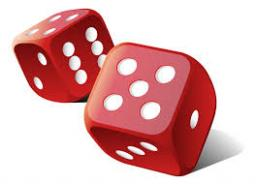Did you find an error or inaccuracy? Feel free to write us. Thank you!Tips to related online calculators
Would you like to compute count of combinations?

## Related math problems and questions:

• DicesWe will throw two dice. What is the probability that the ratio between numbers on first and second dice will be 1:2?
• The diceWhat is the probability of events that if we throw a dice is rolled less than 6?
• ThrowWe throw 2 times with 2 dices. What is the probability that the first roll will fall more than sum of 9 and the second throw have sum 3 or does not have the sum 4?
• One threeWe throw two dice. What is the probability that max one three falls?
• Fall sum or sameFind the probability that if you roll two dice, it will fall the sum of 10, or the same number will fall on both dice.
• Three dice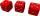What is the probability that when we roll three dice, we roll the numbers 1,2,3?
• DiceWe throw 10 times a playing dice. What is the probability that the six will fall exactly 4 times?
• DiceWe throw five times the dice. What is the probability that six fits exactly twice?
• We rollWe roll two dice A. - what is the probability that the sum of the falling numbers is at most 4 B. - is at least 10 C. - is divisible by 5?
• Sum or productWhat is the probability that two dice fall will have the sum 7 or product 12?
• Six on diceWhat is the probability that when throwing two dice will fall at least one six?
• Three dice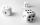When you throw three dice was the sum total of the dice 10. The yellow dice fell one eye more than on the red and brown fell 3 eyes less than red. How many eyes fell on every dice?
• Event probability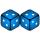The probability of event P in 8 independent experiments is 0.33. What is the probability that the event P occurs in one experiment (chance is the same)?
• Two diceWe roll two dice. What is the probability that the sum of the falling numbers is greater than 3?
• Dice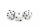How many times must throw the dice, the probability of throwing at least one six was greater than 90%?
• Roll the diceWhat is the probability that if we roll the dice, a number less than 5 falls?
• Guests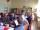How many ways can 9 guests sit down on 10 seats standing in a row?